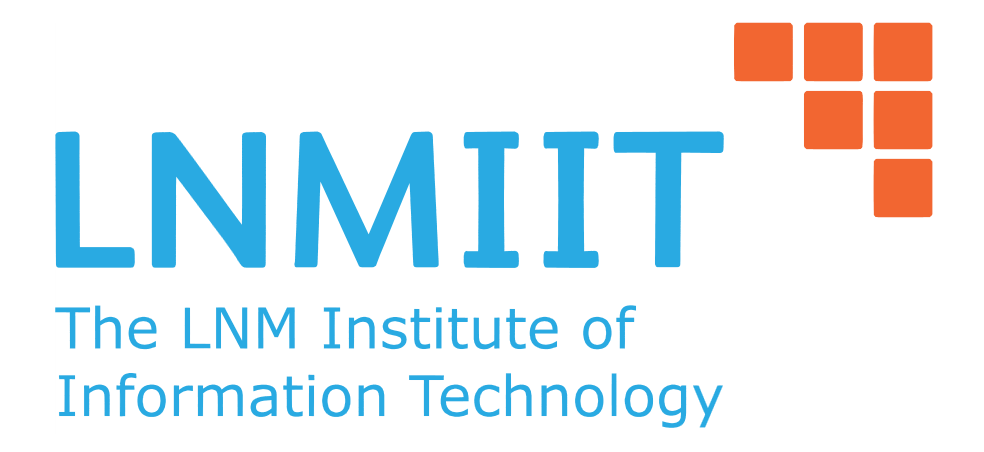Tag(s):

## Dijkstra, Easy-Medium, Graph Theory, Shortest-path, Single Source Shortest Path

Problem
Editorial
Analytics

After very very long time, Pradyumn thought of cleaning his cupboard. As he started to clean his cupboard, he came across a puzzle which he had made it an year ago. But for now, he don’t remember the solution for it. Puzzle consist of a grid like pattern n * m blocks each containing English alphabets(Uppercase and Lowercase). As puzzle was created by Pradyumn, it was not a normal puzzle. This puzzle make self queries, it highlights two places $x_{source}, y_{source}$ and $x_{target}, y_{target}$ in the grid. And, now the player needs to find the shortest distance of reaching from first one to second one. Wait!! Where are distances. So here is the interesting part. Between two adjacent blocks, distance between them is the absolute difference between the ASCII value of characters of the adjacent blocks.
Two blocks are said to be adjacent if they share an edge.
INPUT CONSTRAINTS
$1 \le n \le 10^3$
$1 \le m \le 10^3$
$1 \le x_s,y_s \le 10^3$
$1 \le x_t,y_t \le 10^3$
INPUT FORMAT
Two space separated integers n and m , where n denotes number of rows and m denotes number of columns in the graph
Next n lines of input consist of string having m characters each.
Next two lines contains $x_{source}, y_{source}$ and $x_{target}, y_{target}$ source and target vertex coordinates.
OUTPUT FORMAT
Distance between $x_{source}, y_{source}$ and $x_{target}, y_{target}$, if no such path exist print “Impossible”.

SAMPLE INPUT
2 2
ab
cd
2 2
1 1
SAMPLE OUTPUT
3
Time Limit: 1,0 sec(s) for each input file.
Memory Limit: 256 MB
Source Limit: 1024 KB
Marking Scheme: Marks are awarded when all the testcases pass.
Allowed Languages: Bash, C, C++, C++14, Clojure, C#, D, Erlang, F#, Go, Groovy, Haskell, Java, Java 8, JavaScript(Rhino), JavaScript(Node.js), Julia, Kotlin, Lisp, Lisp (SBCL), Lua, Objective-C, OCaml, Octave, Pascal, Perl, PHP, Python, Python 3, R(RScript), Racket, Ruby, Rust, Scala, Swift, Swift-4.1, TypeScript, Visual Basic

## CODE EDITOR

Initializing Code Editor...

## This Problem was Asked inChallenge Name

LNMIIT Algorithms Cup - June 2017

OTHER PROBLEMS OF THIS CHALLENGE
• Algorithms > Graphs
• Math > Number Theory
• Data Structures > Advanced Data Structures
Уведомления

?